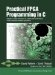# Section 7.4. Limiting Instruction Stages

### 7.4. Limiting Instruction Stages

To get maximum benefit from the optimizer, you should keep in mind those types of statements that will result in a new instruction stage being created. These statements include

• A control statement such as an if test or a loop

• Any access (read or write) to a memory or array that is already being addressed in the current stage

Tip:

To the greatest extent practical, you should reduce the use of unnecessary control statements and memory accesses to reduce the number of instruction stages.

#### Reduce Memory Accesses for Higher Performance

An important consideration when writing your inner code loops for maximum parallelism is to consider data dependencies. In particular, the optimizer will not be capable of parallelizing stages that access the same "bank" of memory (whether expressed as an array or using memory block read and block write functions). For this reason you may want to move subregions of a large array into local storage (local variables or smaller arrays) before performing multiple, otherwise parallel computations on the local data. Doing so will allow the optimizer to parallelize stages more efficiently, with a small trade-off of extra assignments that may be required.

#### Array Splitting

The way that memory (including local arrays) is accessed within a process can have a dramatic impact on the ability of the optimizer to limit instruction stages and to parallelize C statements. Consider the following example:

` x = A + A + A x = x << 2; `

This example involves an array A that is stored in a local RAM block. Only one element of the array can be read from the memory in a single cycle, so the computation must be spread out over four stages:

4. Rerform +A, perform <<2

One way to avoid this problem with memory is to use multiple arrays in multidimensional algorithms. For example, the following algorithm has the same problem as the preceding example:

` int a; for (i=0; i<10; i++) {     a[i] = a[i] + a[i] + a[i]; } `

However, suppose this algorithm is written using a separate array for each row of a, as follows:

` int a0,a1,a2,a3; for (i=0; i<10; i++) {     a3[I] = a0[i] + a1[i] + a2[i]; } `

In this example, each row is stored in a separate block of RAM, allowing each row to be read/written simultaneously. As a result, the body of this loop executes in a single stage instead of the four stages that would be required if the array were not split.

Tip

As this example demonstrates, array splitting is a useful technique to allow multiple simultaneous memory accesses and thereby increase parallelism. In Chapter 10 we'll explore this and other techniques in more detail.Practical FPGA Programming in C
ISBN: 0131543180
EAN: 2147483647
Year: 2005
Pages: 208